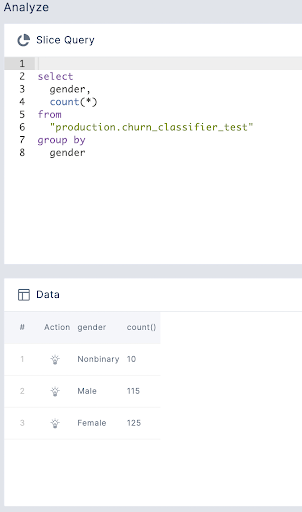# Useful Queries for Root Cause Analysis

This page has an examples of queries which one can use in the Analyze tab to perform Root Cause Analysis of an issue or look at various aspect of the data.

## 1. Count of events from the previous day

In order to look at how many events were published from the previous/last publishing date, we can do it in two ways -

### i. Jump from Monitor tab

This can be done in the following steps -

1. In the monitor tab, click on the 'jump to last event' button to get to the most recent event
2. Select the appropriate time bin, in this case, we can select 1D bin to get day-wise aggregated data
3. Once we have the data in the chart, we can select the most recent bin
4. Select 'Export bin and feature to analyze' to jump to analyze tab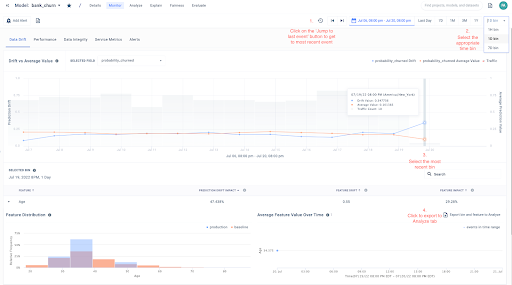1. In the analyze tab, query will be auto-populated based on the Monitor tab selection

2. Modify the query to count the number of events from the selection

``````SELECT
count(*)
FROM
production."bank_churn"
WHERE
fiddler_timestamp BETWEEN '2022-07-20 00:00:00'
AND '2022-07-20 23:59:59'
``````

### ii. Using `date` function in Analyze tab

To know how many events were published on the last publishing day, we can use `date` function of SQL
Use the following query to query number of events

``````select
*
from
"production.churn_classifier_test"
where
date(fiddler_timestamp) = (
select
date(max(fiddler_timestamp))
from
"production.churn_classifier_test"
)
``````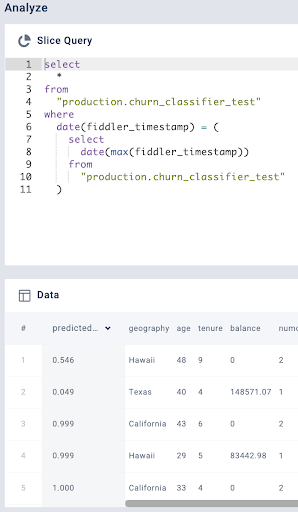## 2. Number of events on last day by output label

If we want to check how many events were published on the last day by the output class, we can use the following query

``````select
churn,
count(*)
from
"production.churn_classifier_test"
where
date(fiddler_timestamp) = (
select
date(max(fiddler_timestamp))
from
"production.churn_classifier_test"
)
group by
churn
``````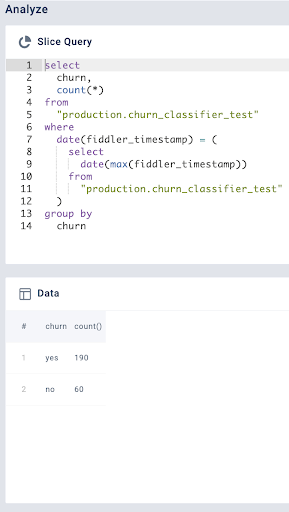## 3. Check events with missing values

If you want to check events where one of the columns is has null values, you can use the `isnull` function.

``````SELECT
*
FROM
production."churn_classifier_test"
WHERE
isnull("estimatedsalary")
LIMIT
1000
``````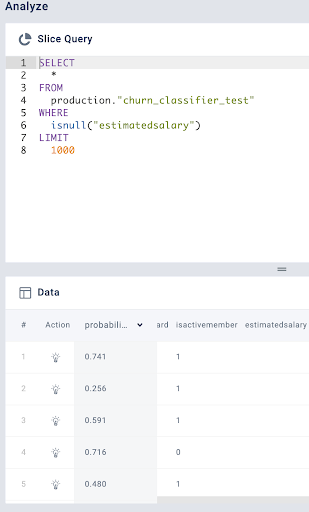## 4. Frequency by Categorical column

We query w.r.t to a categorical field. For example, we can count the number of events by geography which is a categorical column using the following query

``````SELECT
geography,
count(*)
FROM
"production.churn_classifier_test"
GROUP BY
geography

``````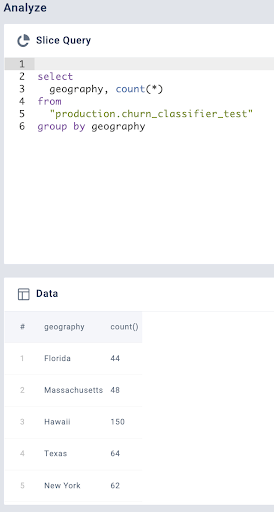We can even query w.r.t to a metadata field. For example, if we consider gender to be a metadata column (specified in ModelInfo object), then we can obtain a frequency of events by the metadata field using the following query

``````SELECT
gender,
count(*)
FROM
"production.churn_classifier_test"
GROUP BY
gender

``````